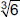International Maths Olympiad Forum By SOF Olympiad Trainer

# User Forum

Subject :IMO    Class : Class 6

## Ans 1:

Class : Class 8
1/2 is the fraction taken by each

Subject :IMO    Class : Class 4

## Ans 1: (Master Answer)

Class : Class 1

Subject :IMO    Class : Class 8

## Ans 1:

Class : Class 8
0 is both a number and the numerical digit used to represent that number in numerals. The number 0 fulfills a central role in mathematics as the additive identity of the integers, real numbers, and many other algebraic structures. As a digit, 0 is used as a placeholder in place value systems.

Subject :IMO    Class : Class 8

## Ans 1:

Class : Class 8
0 is both a number and the numerical digit used to represent that number in numerals. The number 0 fulfills a central role in mathematics as the additive identity of the integers, real numbers, and many other algebraic structures. As a digit, 0 is used as a placeholder in place value systems.

Subject :IMO    Class : Class 8

## Ans 1: (Master Answer)

Class : Class 1

The correct answer is C

We have,Subject :IMO    Class : Class 3

## Ans 1: (Master Answer)

Class : Class 1

1000 in roman numerals = M

Subject :IMO    Class : Class 4

Class : Class 5
Ans. C (20)

Class : Class 5
20

## Ans 3: (Master Answer)

Class : Class 1

The correct answer is C

Number of detective books he read
= 4 × 5 = 20.

Subject :IMO    Class : Class 5

Class : Class 6
B

## Ans 2:

Class : Class 6
(B) 17766 When 17766 is rounded to the nearest tens, it is 17770 When 17766 is rounded to the nearest hundreds, it is 178000 When 17766 is rounded to the nearest thousands, it is 18000 Therefore, 17766 agrees with all the rules and is the right answer.

## Ans 3:

Class : Class 4
Answer is B) 17766

Class : Class 5
B

## Ans 5: (Master Answer)

Class : Class 1

The correct answer is B - 17766

Rounding off rounds off 17766 to the nearest thousand, you will get 18000.

Rounding off 17766  to the nearest hundred, you will get 17800.

And rounding off to the nearest ten, you will get 17770.

## Ans 6:

Class : Class 6
(B) 17766 When 17766 is rounded to the nearest tens, it is 17770 When 17766 is rounded to the nearest hundreds, it is 178000 When 17766 is rounded to the nearest thousands, it is 18000 Therefore, 17766 agrees with all the rules and is the right answer.

Subject :IMO    Class : Class 9

Class : Class 10
D

## Ans 2: (Master Answer)

Class : Class 1

The correct answer is D.Subject :IMO    Class : Class 4

## Ans 1: (Master Answer)

Class : Class 1

The correct answer is D